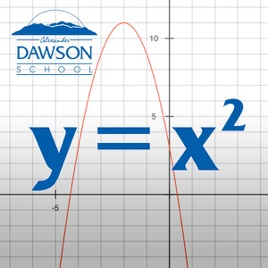17 episódios

Includes files for Chapter 9 from Algebra 1 FUSE app. This chapter focuses on graphing quadratic functions and solving quadratic equations by various techniques including graphing the related function, factoring, square roots, completing the square, and the quadratic formula.# Quadratic Equations and Functions Alexander Dawson School

• Educação

Includes files for Chapter 9 from Algebra 1 FUSE app. This chapter focuses on graphing quadratic functions and solving quadratic equations by various techniques including graphing the related function, factoring, square roots, completing the square, and the quadratic formula.

4.0 de 5
1 avaliação

1 avaliação